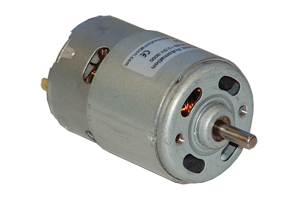# DC MotorDC MotorPhoto Credit - ap-physics.david-s.org

When a voltage is applied to the D.C. Motor, current starts flowing into the positive brush, through the commutator into the armature winding is identical to that of the generator armature winding. Thus conductors under the north field poles carry current in one direction while all conductors under the south field poles carry the current in opposite direction. When the armature carries current it will produce a magnetic field of its own, which will interact with the main field. The result is that there is a force developed on all conductors, tending to turn the armature.
The rotating conductors in a motor armature cut the flux from the poles, so an e.m.f. is developed in them. By Lenz's law this e.m.f. opposes the applied e.m.f. and known as back e.m.f. Eb and is given by mathematical equation
where Φ = Flux per pole
Z = Total number of armature conductors
P = Number of Poles
A = Number of parallel paths in armature
N = Speed of rotation

#### Fundamental Voltage

Fundamental voltage equation of motor is expressed by
where Eb = Back e.m.f.
V = Potential difference applied to the brushes in volts
Ia = Armature Current
R= Armature circuit Resistance